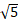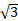# GSEB Solutions for ધોરણ ૧૧ Gujarati

#### GSEB std 10 science solution for Gujarati check Subject Chapters Wise::

(5i) (-i) આપેલ સંકર સંખ્યાને a + ib સ્વરૂપમાં રૂપાંતર કરો. (સ્વાધ્યાય 5.1)

જવાબ : (5i) (-i) = -3i2                    = -3 (-1)                    = 3                    = 3 + 0 . i

i9 + i19 આપેલ સંકર સંખ્યાને a + ib સ્વરૂપમાં રૂપાંતર કરો. (સ્વાધ્યાય 5.1)

જવાબ : i9 + i19 = i8+1 + i16+3                 = i4´2 . i + i4´4 . i3                 = i + i3      ( i4k = 1)                 = i + i2      ( i2 = -1)
= i + (-1)i
= i - i                 = 0                 = 0 + 0 . i

i-39 આપેલ સંકર સંખ્યાને a + ib સ્વરૂપમાં રૂપાંતર કરો. (સ્વાધ્યાય 5.1)

જવાબ :3(7 + i7) + i(7 + i7) આપેલ સંકર સંખ્યાને a + ib સ્વરૂપમાં રૂપાંતર કરો. (સ્વાધ્યાય 5.1)

જવાબ : 3(7 + i 7) + i(7 + i 7) = 21 + i . 21 + 7i + 7 . i2 = 21 + 28 . i - 7  ( i2 = -1) = 14 + 28 i

(1 - i) - (-1 + i6) આપેલ સંકર સંખ્યાને a + ib સ્વરૂપમાં રૂપાંતર કરો. (સ્વાધ્યાય 5.1)

જવાબ : (1 - i) - (-1 + i6) = 1 - i + 1 - 6i = 2 - 7iજવાબ :જવાબ :(1 - i)4 આપેલ સંકર સંખ્યાને a + ib સ્વરૂપમાં રૂપાંતર કરો. (સ્વાધ્યાય 5.1)

જવાબ : (1 - i)4 = [(1 - i)2]2 = [1 - 2i + i2]2 = [1 - 2i - 1]2     ( i2 = -1) = [-2i]2 = 4i2 = -4 = -4 + 0. i

(+ 3i)3 આપેલ સંકર સંખ્યાને a + ib સ્વરૂપમાં રૂપાંતર કરો. (સ્વાધ્યાય 5.1)

જવાબ :જવાબ :4 - 3i આપેલ સંકર સંખ્યાનો ગુણાકારની વ્યસ્ત સંખ્યા મેળવો. (સ્વાધ્યાય 5.1)

જવાબ :+ 3i આપેલ સંકર સંખ્યાનો ગુણાકારની વ્યસ્ત સંખ્યા મેળવો. (સ્વાધ્યાય 5.1)

જવાબ :-i આપેલ સંકર સંખ્યાનો ગુણાકારની વ્યસ્ત સંખ્યા મેળવો. (સ્વાધ્યાય 5.1)

જવાબ :જવાબ :z = -1 - iઆપેલ સંકર સંખ્યાનો માનાંક અને કોણાંક મેળવો. (સ્વાધ્યાય 5.2)

જવાબ :z = -+ i આપેલ સંકર સંખ્યાનો માનાંક અને કોણાંક મેળવો. (સ્વાધ્યાય 5.2)

જવાબ :1 - i આપેલ સંકર સંખ્યાને ધ્રુવીય સ્વરૂપમાં રૂપાંતરિત કરો. (સ્વાધ્યાય 5.2)

જવાબ :-1 + i આપેલ સંકર સંખ્યાને ધ્રુવીય સ્વરૂપમાં રૂપાંતરિત કરો. (સ્વાધ્યાય 5.2)

જવાબ :-1 - i આપેલ સંકર સંખ્યાને ધ્રુવીય સ્વરૂપમાં રૂપાંતરિત કરો. (સ્વાધ્યાય 5.2)

જવાબ :-3 આપેલ સંકર સંખ્યાને ધ્રુવીય સ્વરૂપમાં રૂપાંતરિત કરો. (સ્વાધ્યાય 5.2)

જવાબ :+ i આપેલ સંકર સંખ્યાને ધ્રુવીય સ્વરૂપમાં રૂપાંતરિત કરો. (સ્વાધ્યાય 5.2)

જવાબ :i આપેલ સંકર સંખ્યાને ધ્રુવીય સ્વરૂપમાં રૂપાંતરિત કરો. (સ્વાધ્યાય 5.2)

જવાબ :-15 - 8i આપેલ સંખ્યાનું વર્ગમૂળ મેળવો. (સ્વાધ્યાય 5.4)

જવાબ :-8 - 6i આપેલ સંખ્યાનું વર્ગમૂળ મેળવો. (સ્વાધ્યાય 5.4)

જવાબ :1 - i આપેલ સંખ્યાનું વર્ગમૂળ મેળવો. (સ્વાધ્યાય 5.4)

જવાબ :i આપેલ સંખ્યાનું વર્ગમૂળ મેળવો. (સ્વાધ્યાય 5.4)

જવાબ :1 + i આપેલ સંખ્યાનું વર્ગમૂળ મેળવો. (સ્વાધ્યાય 5.4)

જવાબ :